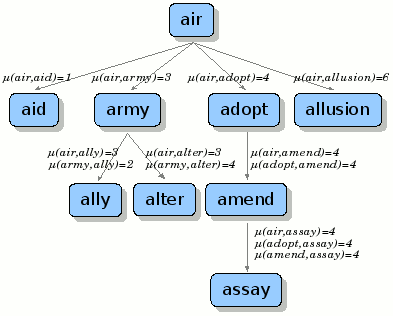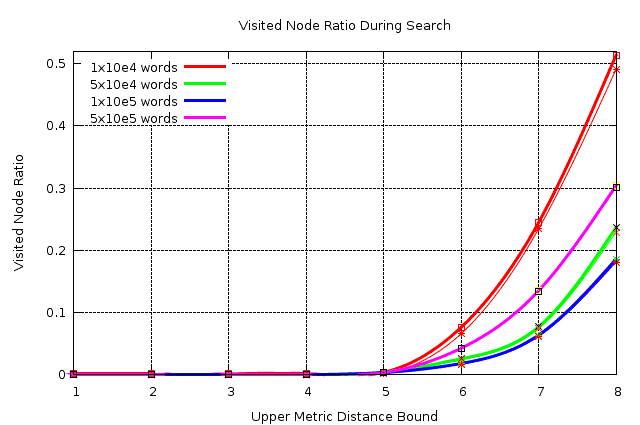## bk-tree

2013-04-20

No Description

### Upstream URL

github.com/vy/bk-tree

Not determined

``````     ___           ___
/  /\         /  /\
/  /::\       /  /:/
/  /:/\:\     /  /:/
/  /::\ \:\   /  /::\____
/__/:/\:\_\:| /__/:/\:::::\
\  \:\ \:\/:/ \__\/~|:|~~~~              ___           ___           ___
\  \:\_\::/     |  |:|    ___          /  /\         /  /\         /  /\
\  \:\/:/      |  |:|   /__/\        /  /::\       /  /::\       /  /::\
\__\::/       |__|:|   \  \:\      /  /:/\:\     /  /:/\:\     /  /:/\:\
~~         \__\|    \__\:\    /  /::\ \:\   /  /::\ \:\   /  /::\ \:\
/  /::\  /__/:/\:\_\:\ /__/:/\:\ \:\ /__/:/\:\ \:\
/  /:/\:\ \__\/~|::\/:/ \  \:\ \:\_\/ \  \:\ \:\_\/
/  /:/__\/    |  |:|::/   \  \:\ \:\    \  \:\ \:\
/__/:/         |  |:|\/     \  \:\_\/     \  \:\_\/
\__\/          |__|:|~       \  \:\        \  \:\
\__\|         \__\/         \__\/
``````

This program implements a derivative of BK-Tree data structure described in "Some Approaches to Best-Match File Searching" paper of W. A. Burkhard and R. M. Keller. For more information about the paper, see

``````@article{362025,
author = {W. A. Burkhard and R. M. Keller},
title = {Some approaches to best-match file searching},
journal = {Commun. ACM},
volume = {16},
number = {4},
year = {1973},
issn = {0001-0782},
pages = {230--236},
doi = {http://doi.acm.org/10.1145/362003.362025},
publisher = {ACM},
address = {New York, NY, USA},
}
``````

In the implementation, I have used below structure to store values in the nodes:

``````struct node {
distance: Metric distance between current node and its parent.
value   : Value stored in current node.
nodes   : Nodes collected under this node.
}
``````

See below figure for an example.During every search phase, instead of walking through nodes via

``````j = {j, j+1, j-1, j+2, j-2, ...}
= {0, (-1)^i+1 * ceil(i/2)}, i = 1, 2, 3, ...
``````

as described in the original paper, program sorts nodes according their relative distance to value being searched:

``````distance = d(searched-value, current-node-value)
sort(nodes, lambda(node) { abs(distance - distance-of(node)) }
``````

There is no restriction on the type of the value which will be stored in the tree, as long as you supply appropriate metric function.

# Performance

Here is the results of a detailed test performed using `BK-TREE` package.

In every test, 100 random words are searched in the randomly created word databases. Words stored in the database are varying from 5 characters upto 10 characters.

DB Size (words)Threshold (distance)Scanned Node %Found Node %
10,00010.1100.0100
20.1100.0100
30.1100.0100
40.1600.0300
50.3700.1100
67.6006.5800
724.46023.4300
851.36049.0900
50,00010.00220.0020
20.00230.0021
30.02510.0030
40.04080.0127
50.39430.3196
62.54302.3869
77.68767.3874
823.663522.9339
100,00010.00120.0010
20.00120.0011
30.00130.0017
40.02310.0085
50.33830.2998
61.79571.7079
76.35716.1654
818.559918.0996
500,00010.00270.0002
20.00290.0002
30.00390.0011
40.00120.0081
50.34440.3213
60.42440.4201
713.483413.3619
830.372830.1665

How this table should be interpreted? The lower the difference between the third and fourth columns, the less redundant node visit performed. And the stability of this difference (which means no fluctuations in the difference) indicates the stability of the convergence.

Here is the graph of above results.# Example

Here is an example about how to used supplied interface.

``````(defpackage :bk-tree-test (:use :cl :bk-tree))

(in-package :bk-tree-test)

(defvar *words* nil)

(defvar *tree* (make-instance 'bk-tree))

;; Build *WORDS* list.
(with-open-file (in "/home/vy/lisp/english-words.txt")
(loop for line = (read-line in nil nil)
while line
do (push
(string-trim '(#\space #\tab #\cr #\lf) line)
*words*)))

;; Check *WORDS*.
(if (endp *words*)
(error "*WORDS* is empty!"))

;; Fill the *TREE*.
(mapc
(lambda (word)
(handler-case (insert-value word *tree*)
(duplicate-value (ctx)
(format t "Duplicated: ~a~%" (value-of ctx)))))
*words*)

;; Let's see that green tree.
(print-tree *tree*)

;; Test BK-Tree.
(time
(mapc
(lambda (result)
(format t "~a ~a~%" (distance-of result) (value-of result)))
(search-value "kernel" *tree* :threshold 2)))

;; Test brute levenshtein.
(time
(loop with target-word = "kernel"
with results = (sort
(mapcar
(lambda (word)
(cons (levenshtein target-word word) word))
*words*)
#'<
:key #'car)
repeat 50
for (distance . value) in results
while (<= distance 2)
do (format t "~a ~a~%" distance value)))``````

# Caveats

For performance reasons, `LEVENSHTEIN` function coming with the package has some limitations both on the input string and penalty costs.

``````(deftype levenshtein-cost ()
"Available penalty costs."
'(integer 0 7))

(deftype levenshtein-input-length ()
"Maximum distance a comparison can result."
`(integer 0 ,(- most-positive-fixnum 7)))``````

Just in case, configure these variables suitable to your needs.

## Dependents (0)

• GitHub
• Quicklisp